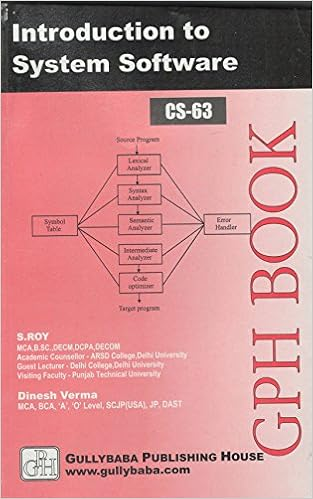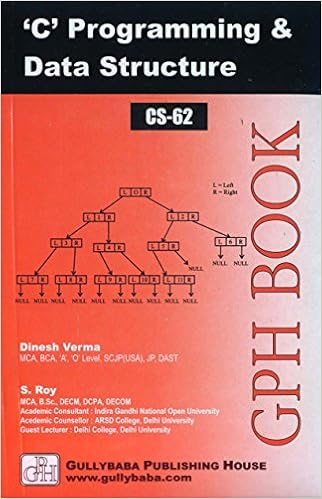IGNOU BCA MCA.comQuestion Papers of IGNOU MCA-OLD CS08

 CS08: Numerical & Statical ComputingYear: 1999 TEE: june Time 3 Full Marks 75 Note: Question 1 is compulsory. Answer any three from the rest.Q.1(a): Do as directed Q.1(a)(: Write FORTRAN logical expression to test the condition that either the variable H is greater than 5 or the value of the variable K is not zero.: Q.1(a)(: Given FORTAN program segment DO 10 I = 1, 510 A(I) = 6 - 1Write equivalent program segment using IF and GOTO statements but not using DO statement.: Q.1(a)(: Given that B is a 5 X 4 matrix, explain the meaning of the following FORTRAN statement READ (5, 200) ((B(I, J), I = 1, 5) J = 1, 4): home || Next >>